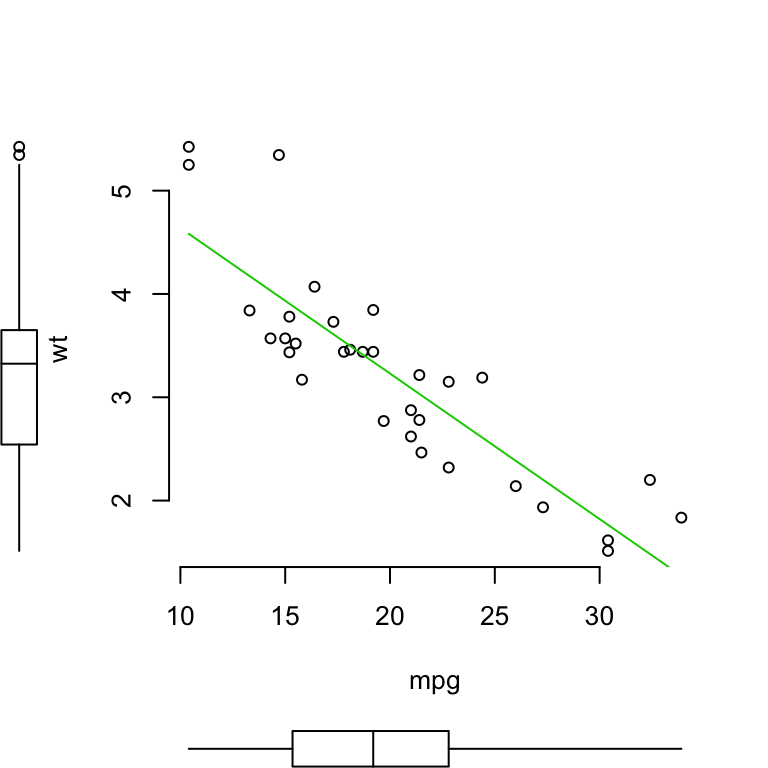# Scatter Plots - R Base Graphs

Previously, we described the essentials of R programming and provided quick start guides for importing data into R.

Here, we’ll describe how to make a scatter plot. A scatter plot can be created using the function plot(x, y). The function lm() will be used to fit linear models between y and x. A regression line will be added on the plot using the function abline(), which takes the output of lm() as an argument. You can also add a smoothing line using the function loess().

1. Launch RStudio as described here: Running RStudio and setting up your working directory

2. Prepare your data as described here: Best practices for preparing your data and save it in an external .txt tab or .csv files

3. Import your data into R as described here: Fast reading of data from txt|csv files into R: readr package.

Here, we’ll use the R built-in mtcars data set.

# R base scatter plot: plot()

``````x <- mtcars\$wt
y <- mtcars\$mpg
# Plot with main and axis titles
# Change point shape (pch = 19) and remove frame.
plot(x, y, main = "Main title",
xlab = "X axis title", ylab = "Y axis title",
pch = 19, frame = FALSE)
plot(x, y, main = "Main title",
xlab = "X axis title", ylab = "Y axis title",
pch = 19, frame = FALSE)
abline(lm(y ~ x, data = mtcars), col = "blue")````````````# Add loess fit
plot(x, y, main = "Main title",
xlab = "X axis title", ylab = "Y axis title",
pch = 19, frame = FALSE)
lines(lowess(x, y), col = "blue")``````# Enhanced scatter plots: car::scatterplot()

The function scatterplot() [in car package] makes enhanced scatter plots, with box plots in the margins, a non-parametric regression smooth, smoothed conditional spread, outlier identification, and a regression line, …

• Install car package:
``install.packages("car")``
• Use scatterplot() function:
``````library("car")
scatterplot(wt ~ mpg, data = mtcars)``````The plot contains:

• the points
• the regression line (in green)
• the smoothed conditional spread (in red dashed line)
• the non-parametric regression smooth (solid line, red)

``````# Suppress the smoother and frame
scatterplot(wt ~ mpg, data = mtcars,
smoother = FALSE, grid = FALSE, frame = FALSE)````````````# Scatter plot by groups ("cyl")
scatterplot(wt ~ mpg | cyl, data = mtcars,
smoother = FALSE, grid = FALSE, frame = FALSE)``````It’s also possible to add labels using the following arguments:

• labels: a vector of point labels
• id.n, id.cex, id.col: Arguments for labeling points specifying the number, the size and the color of points to be labelled.

``````# Add labels
scatterplot(wt ~ mpg, data = mtcars,
smoother = FALSE, grid = FALSE, frame = FALSE,
labels = rownames(mtcars), id.n = nrow(mtcars),
id.cex = 0.7, id.col = "steelblue",
ellipse = TRUE)````````````##           Mazda RX4       Mazda RX4 Wag          Datsun 710      Hornet 4 Drive   Hornet Sportabout             Valiant
##                   1                   2                   3                   4                   5                   6
##          Duster 360           Merc 240D            Merc 230            Merc 280           Merc 280C          Merc 450SE
##                   7                   8                   9                  10                  11                  12
##          Merc 450SL         Merc 450SLC  Cadillac Fleetwood Lincoln Continental   Chrysler Imperial            Fiat 128
##                  13                  14                  15                  16                  17                  18
##         Honda Civic      Toyota Corolla       Toyota Corona    Dodge Challenger         AMC Javelin          Camaro Z28
##                  19                  20                  21                  22                  23                  24
##    Pontiac Firebird           Fiat X1-9       Porsche 914-2        Lotus Europa      Ford Pantera L        Ferrari Dino
##                  25                  26                  27                  28                  29                  30
##       Maserati Bora          Volvo 142E
##                  31                  32``````

Other arguments can be used such as:

• log to produce log axes. Allowed values are log = “x”, log = “y” or log = “xy”
• boxplots: Allowed values are:
• “x”: a box plot for x is drawn below the plot
• “y”: a box plot for y is drawn to the left of the plot
• “xy”: both box plots are drawn
• “” or FALSE to suppress both box plots.
• ellipse: if TRUE data-concentration ellipses are plotted.

# 3D scatter plots

To plot a 3D scatterplot the function scatterplot3D [in scatterplot3D package can be used].

The following R code plots a 3D scatter plot using iris data set.

``head(iris)``
``````##   Sepal.Length Sepal.Width Petal.Length Petal.Width Species
## 1          5.1         3.5          1.4         0.2  setosa
## 2          4.9         3.0          1.4         0.2  setosa
## 3          4.7         3.2          1.3         0.2  setosa
## 4          4.6         3.1          1.5         0.2  setosa
## 5          5.0         3.6          1.4         0.2  setosa
## 6          5.4         3.9          1.7         0.4  setosa``````
``````# Prepare the data set
x <- iris\$Sepal.Length
y <- iris\$Sepal.Width
z <- iris\$Petal.Length
grps <- as.factor(iris\$Species)
# Plot
library(scatterplot3d)
scatterplot3d(x, y, z, pch = 16)````````````# Change color by groups
# add grids and remove the box around the plot
# Change axis labels: xlab, ylab and zlab
colors <- c("#999999", "#E69F00", "#56B4E9")
scatterplot3d(x, y, z, pch = 16, color = colors[grps],
grid = TRUE, box = FALSE, xlab = "Sepal length",
ylab = "Sepal width", zlab = "Petal length")``````# Summary

Create a scatter plot:

• Using R base function:
``with(mtcars, plot(wt, mpg, frame = FALSE))``
• Using car package:
``````car::scatterplot(wt ~ mpg, data = mtcars,
smoother = FALSE, grid = FALSE)``````
• 3D scatter plot:
``````library(scatterplot3d)
with(iris,
scatterplot3d(x = Sepal.Length, y = Sepal.Width,
z = Petal.Length, pch = 16,
grid = TRUE, box = FALSE)
)``````

# Infos

This analysis has been performed using R statistical software (ver. 3.2.4).